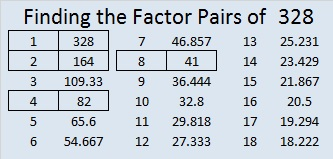# 328 and Level 6

• 328 is a composite number.
• Prime factorization: 328 = 2 x 2 x 2 x 41, which can be written (2^3) x 41
• The exponents in the prime factorization are 3 and 1. Adding one to each and multiplying we get (3 + 1)(1 + 1) = 4 x 2 = 8. Therefore 328 has exactly 8 factors.
• Factors of 328: 1, 2, 4, 8, 41, 82, 164, 328
• Factor pairs: 328 = 1 x 328, 2 x 164, 4 x 82, or 8 x 41
• Taking the factor pair with the largest square number factor, we get √328 = (√4)(√82) = 2√82 ≈ 18.111Will these 13 clues in this puzzle stump you?Print the puzzles or type the factors on this excel file: 12 Factors 2014-12-15## One thought on “328 and Level 6”

1.Joseph Nebus

Well, it’s hard to set up a tree so it stays perfectly vertical.

This site uses Akismet to reduce spam. Learn how your comment data is processed.